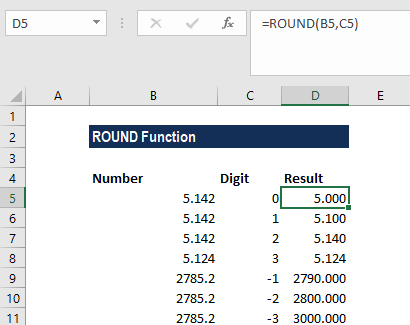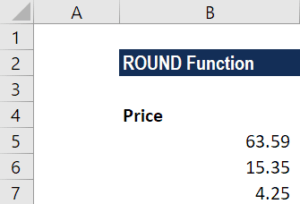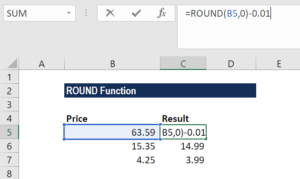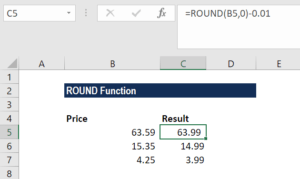# ROUND Function

Rounds up a number to a specified number of digits

## What is the ROUND Function?

The ROUND Function is categorized under Excel Math and Trigonometry functions. The function will round up a number to a specified number of digits. Unlike the ROUNDUP and ROUNDDOWN functions, the ROUND function can round either up or down.

As a financial analyst, this function is useful as it helps round a number and eliminates the least significant digits, simplifying the notation but keeping close to the original value.

### Formula

=ROUND(number,num_digits)

The function uses the following arguments:

1. Number (required argument) – This is a real number that we wish to round.
2. Num_digits (required argument) – This is the number of digits to which we want to round the number.

Now if the num_digits argument is:

1. A positive value that is greater than zero, it specifies the number of digits to the right of the decimal point.
2. Equal to zero, it specifies rounding to the nearest integer.
3. A negative value that is less than zero, it specifies the number of digits to the left of the decimal point.

### How to use the ROUND Function in Excel?

To understand the uses of the ROUND function, let’s consider a few examples:

#### Example 1

Suppose we are given the following data:

NumberDigitsRemarks
5.1420Round up to the nearest whole number
5.1421Round up to 1 decimal place
5.1422Round up to 2 decimal places
2785.23Round up to 3 decimal places
2785.2-1Round up to the nearest 10
2785.2-2Round up to the nearest 100
2785.2-3Round up to the nearest 1000

The results we get in Excel are shown below:#### Example 2

Let’s see how to round prices using the function to the nearest 0.99 value. Suppose we are given the following data:The formula used is shown below:The ROUND function would first round 63.39 to 63 and then subtract 0.01 to give 62.99. So, the function with a zero would round the number given to the nearest whole dollar. Once it is rounded, the formula would simply subtract 0.01 from the number to get a .99 value.

We get the result below:Thanks for reading CFI’s guide to the Excel ROUND function. By taking the time to learn and master these Excel functions, you’ll significantly speed up your financial analysis. To learn more, check out these additional CFI resources:

• Excel Functions for Finance